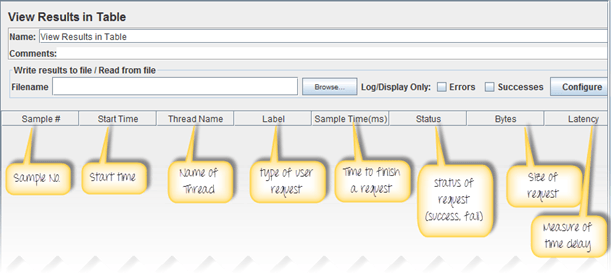### What are Timers?

By default, JMeter sends the request without pausing between each request. In that case, JMeter could overwhelm your test server by making too many requests in a short amount of times.

Let imagine that you send thousands request to a web server under test in a few seconds. This is what happens!

Timers allow JMeter to delay between each request which a thread makes. A timer can solve the server overload problem.

Also, in real life visitors do not arrive at a website all at the same time, but at different time intervals. So Timer will help mimic the real-time behavior.

In this tutorial, you will learn

Following are some common types of a timer in JMeter

## Constant Timer:

Constant timer delays each user request for the same amount of time.

## Gaussian Random Timer:

Gaussian random timer delays each user request for a random amount of time.

### Parameters:

Attribute Description
Name Descriptive name for this timer that is shown in the tree
Deviations (milliseconds) A parameter of Gaussian Distribution Function
Constant Delay Offset (milliseconds) Additional value in milliseconds

So the total delay is described as below figure:

## Uniform Random Timer:

Uniform random timer delays each user request for a random amount of time.

### Parameters:

Attribute

Description

Name

Descriptive name for this timer that is shown in the tree

Random Delay Maximum

Maximum random number of milliseconds to delay.

Constant Delay Offset (milliseconds)

The total delay is the sum of the random value and the offset value.

## BeanShell Timer

The BeanShell Timer can be used to generate a delay time between each user request.

## BSF Timer

The BSF Timer can be used to generate a delay between each user request using a BSF scripting language.

## JSR223 Timer

The JSR223 Timer can be used to generate a delay between each user request using a JSR223 scripting language

## How to Use Constant Timer

In this example, you will use Constant Timer to set a fixed delay between user requests to google.com.

1. JMeter creates one user request to http://www.google.com 100 times
2. Delay between each user request is 5000 ms

Here is the roadmap for this practical example:

## Pre-condition:

We re-use the Step 1 and Step 2 in tutorial JMeter Performance Testing.

This setting lets JMeter create one user request to http://www.google.com in 100 times

### Step 3) Add Constant Timer

Right-click Thread Group -> Timer -> Constant Timer

Configuring Thread Delay of 5000 milliseconds

### Step 4) Add View Results in Table

View Results in Table displays the test result in table format.

Right click Add -> Listener -> View Result in Table

View Results in Table displays as below figure### Step 5) Run your test

When you ready to run a test, click the Run button on the menu bar, or short key Ctrl+R

This is the result of this test

For example, in the above figure, let analyze the Sample 2

• Start time is 22:05:01.866
• Sample Time of Sample 2 is 172 ms
• Constant Timer: 5000 ms (as configured)
• End Time of this sample is = 22:05:01.866 + 172 + 5000 = 22:05:07.038

So the Sample 3 should start at the time is 22:05:07.039 ( As shown in the above figure)

The delay of each sample is 5000 ms

If you change the Constant Timer is zero, you will see the result is changed

Let analyze the Sample 1

• Start time is 22:17:39.141
• Sample Time of Sample 2 is 370 ms
• Constant Timer : 0 ms (as configured)
• End Time of this sample is = 22:17:39.141+ 370 + 0 = 22:17:39.511

So the Sample 2 should start at the time is 22:17:39.512 (Shown in the above figure)

## Troubleshooting:

If you face the issue while running the above scenario ... do the following

1. Check whether you are connecting to the internet via a proxy. If yes, remove the proxy.
2. Open a new instance of JMeter
3. Open the TimerTestPlan.jmx in Jmeter
4. Double Click on Thread Group -> View Result in Table
5. Run the Test

YOU MIGHT LIKE: